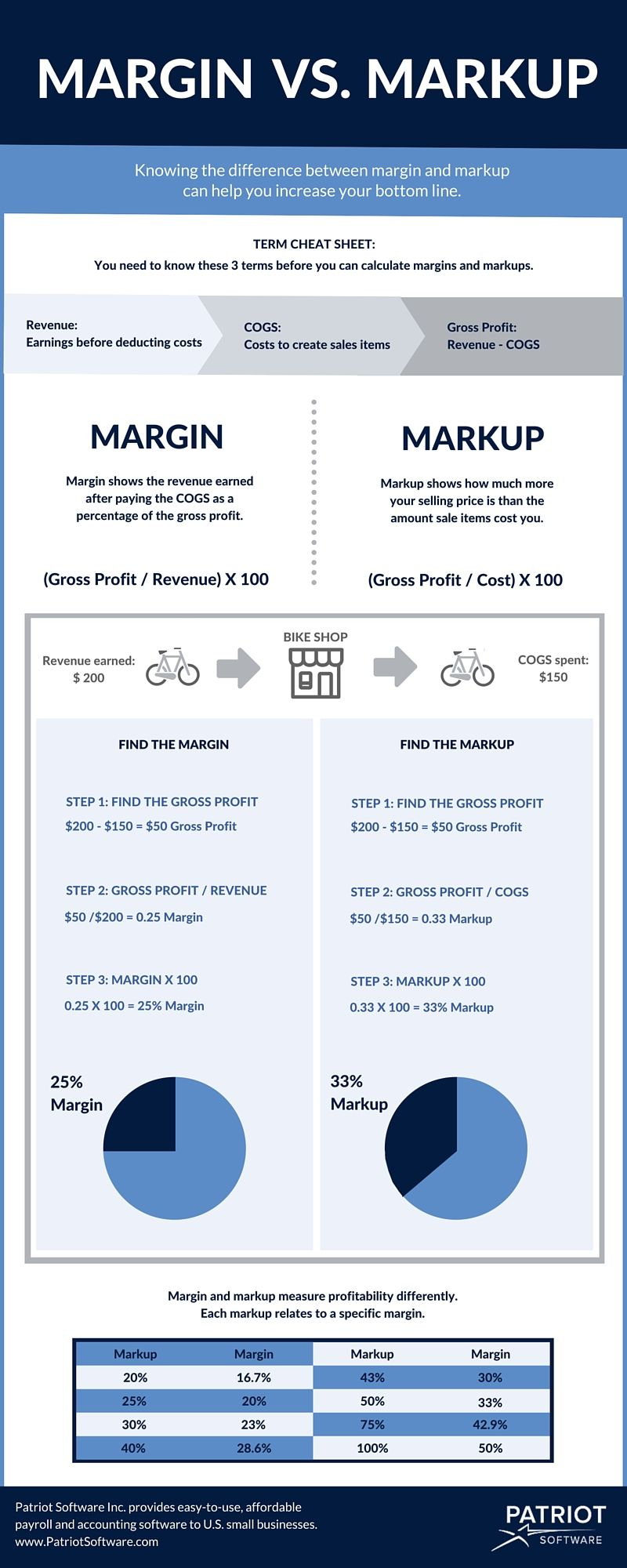Markup vs. Margin Chart & Infographic | Calculating Margin & Markup
We are committed to providing timely updates regarding COVID-19.

# Markup vs. Margin Chart: How to Calculate Margin and Markup

Business owners often confuse margin and markup. Both figures help you set prices and measure productivity. But, a margin vs. markup chart shows that the two terms reflect profit differently.

It’s important to know the difference between margins and markups in accounting. Read on to learn about markup vs. margin. And, don’t forget to check out our infographic at the bottom of this page.

## Terms to help understand margin and markup

To understand margin vs. markup, first know these three terms:

• Revenue
• Cost of goods sold (COGS)
• Gross profit

Revenue is the income you earn by selling your products and services. Revenue is the top line of your income statement and reflects earnings before deductions.

Cost of goods sold (COGS) includes the expenses that go into making your products and providing your services. Calculating COGS could include adding up materials and direct labor costs.

Gross profit is the revenue left over after you pay the expenses of making your products and providing your services. Gross profit is revenue minus COGS.

You will use these three terms when finding both margin and markup. Understanding the terms will help you grasp the difference between margin and markup.

## Markup vs. margin

Calculating margin and markup is key to setting prices that not only cover your expenses but also leave you with a profit.

Learn the difference between margin vs. markup below.

### How to calculate margin

A margin, or gross margin, shows the revenue you make after paying COGS. To calculate margin, start with your gross profit (Revenue – COGS). Then, find the percentage of the revenue that is gross profit. You can find the percentage of revenue that is gross profit by dividing your gross profit by revenue.

For example, you sell bicycles for \$200 each. Each bicycle costs you \$150. First, find your gross profit, or the difference between the revenue (\$200) and the cost (\$150).

\$200 – \$150 = \$50 gross profit

To find the margin, divide gross profit by the revenue.

\$50 / \$200 = 0.25 margin

To make the margin a percentage, multiply the result by 100.

0.25 X 100 = 25% margin

The margin is 25%. That means you keep 25% of your total revenue. You spent the other 75% of your revenue on buying the bicycle.

The margin formula measures how much of every dollar in sales you keep after paying expenses. In the margin calculation example above, you keep \$0.25 for every dollar you make. The greater the margin, the greater the percentage of revenue you keep when you make a sale.

### How to calculate markup

Markups are different than margins. A markup shows how much more your selling price is than the amount the item costs you.

Like a margin, you start finding a markup with your gross profit (Revenue – COGS). Then, find the percentage of the COGS that is gross profit. You can find this percentage by dividing your gross profit by COGS.

Using the bicycle example from above, you sell each bicycle for \$200. The bicycle costs you \$150. First, find the gross profit.

\$200 – \$150 = \$50 gross profit

To write the markup as a percentage, divide the gross profit by the COGS.

\$50 / \$150 = 0.33 markup

To make the markup a percentage, multiply the result by 100.

0.33 X 100 = 33% markup

The markup is 33%. That means you sold the bicycle for 33% more than the amount you paid for it.

The markup formula measures how much more you sell your items for than the amount you pay for them. In the markup example above, your markup is 33%. The higher the markup, the more revenue you keep when you make a sale.## How to convert markup to margin and margin to markup

There may come a time when you know your margin and want to convert it to get your markup. Likewise, you might know your markup and want to find your margin.

### Margin to markup conversion

To convert margin into markup, use the following formula:

Markup = [Margin / (1 – Margin)] X 100

Let’s say you want a 30% margin and want to know how much your markup should be. You would do:

Markup = [0.30 / (1 – .30)] X 100

Markup = 43%

### Markup to margin conversion

Now, to convert markup to margin, use this formula:

Margin = [Markup / (1+ Markup)] X 100

Say you want a markup of 50% and would like to know how much your margin is.  You would do:

Margin = [0.50 / (1 + 0.50) X 100

Margin = 33%

## Margin vs. markup chart

Margins and markups interact in a predictable way. Each markup relates to a specific margin and vice versa. Markups are always higher than their corresponding margins.

To easily find the markups that correlate to margins, use this margin vs. markup chart:

Markup Margin
15% 13%
20% 16.7%
25% 20%
30% 23%
33.3% 25%
40% 28.6%
43% 30%
50% 33%
75% 42.9%
100% 50%

## Why margin vs. markup matters

Knowing the difference between a markup and a margin helps you set goals. If you know how much profit you want to make, you can set your prices accordingly using the margin vs. markup formulas.

If you don’t know your margins and markups, you might not know how to price a product or service correctly. This could cause you to miss out on revenue. Or, you might be asking too much, and many potential customers are not willing to pay your prices.

Check your margins and markups often to be sure you’re getting the most out of your strategic pricing.

## Markup vs. margin: Ultimate infographic

Still need help with margin vs. markup? Check out our infographic to learn more.